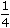# simplification aptitude questions

6. A sum of Rs. 1360 has been divided among A, B and C such that A getsof what B gets and B getsof what C gets. B’s share is:
 A. Rs. 120 B. Rs. 160 C. Rs. 240 D. Rs. 300

Explanation:

Let C’s share = Rs. x

 Then, B’s share = Rs. x ,   A’s share = Rs.2 x x= Rs. x 4 3 4 6x + x + x = 1360 6 417x = 1360 12x = 1360 x 12 = Rs. 960 17

 Hence, B’s share = Rs.960= Rs. 240. 4

7. One-third of Rahul’s savings in National Savings Certificate is equal to one-half of his savings in Public Provident Fund. If he has Rs. 1,50,000 as total savings, how much has he saved in Public Provident Fund ?
 A. Rs. 30,000 B. Rs. 50,000 C. Rs. 60,000 D. Rs. 90,000

Explanation:

Let savings in N.S.C and P.P.F. be Rs. x and Rs. (150000 – x) respectively. Then,

 1 x = 1 (150000 – x) 3 2x + x = 75000 3 25x = 75000 6x = 75000 x 6 = 90000 5Savings in Public Provident Fund = Rs. (150000 – 90000) = Rs. 60000

8. A fires 5 shots to B’s 3 but A kills only once in 3 shots while B kills once in 2 shots. When B has missed 27 times, A has killed:
 A. 30 birds B. 60 birds C. 72 birds D. 90 birds

Explanation:

Let the total number of shots be x. Then,

 Shots fired by A = 5 x 8

 Shots fired by B = 3 x 8

 Killing shots by A = 1 of 5 x = 5 x 3 8 24

 Shots missed by B = 1 of 3 x = 3 x 2 8 163x = 27 or x =27 x 16= 144. 16 3

 Birds killed by A = 5x =5 x 144= 30. 24 24

9. Eight people are planning to share equally the cost of a rental car. If one person withdraws from the arrangement and the others share equally the entire cost of the car, then the share of each of the remaining persons increased by:
A.
 1 7
B.
 1 8
C.
 1 9
D.
 7 8

Explanation:

 Original share of 1 person = 1 8

 New share of 1 person = 1 7

 Increase =1 – 1= 1 7 8 56Required fraction = (1/56) =1 x 8= 1 (1/8) 56 1 7

10. To fill a tank, 25 buckets of water is required. How many buckets of water will be required to fill the same tank if the capacity of the bucket is reduced to two-fifth of its present ?
 A. 10 B. 35 C. 62.5 D. Cannot be determined E. None of these

Explanation:

Let the capacity of 1 bucket = x.

Then, the capacity of tank = 25x.

 New capacity of bucket = 2 x 5Required number of buckets = 25x (2x/5)

 =25x x 52x

 = 125 2

= 62.5